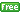www.gllamm.org

## How do I fit a latent class model?

 Title Fitting latent class models in gllamm Author Sophia Rabe-Hesketh, University of California, Berkeley Date July 2012

### Exploratory latent class model for binary variables

In an exploratory latent class model for I binary variables yij for units j, each unit is assumed to belong to one of C latent classes c with probability πc. Each latent class has a different probability pi|c that the ith variable takes the value 1. Given latent class membership, the variables yij are conditionally independent. The marginal probabilities are then

P(yij=1) = ∑c πcpi|c

(The sum over the latent classes of the probability that the subject belongs to that latent class times the latent-class-specific probability that the variable is 1.)

### Brief explanation of estimation in gllamm

#### Data preparation

As always, the data must be in long form, with all yij in one variable `y` and with variables `i` and `j` keeping track of the variable and unit identifiers, i and j, respectively.

#### Parameterization in gllamm and syntax

In `gllamm`, we treat eic=logit[pi|c] as the C discrete values that I latent variables can take. There is one latent variable ηij for each response variable yij. The vector ηj of latent variables for subject j takes the values ec (with elements eic) if subject j is in latent class c. Such a discrete latent variable distribution, with associated probabilities πc, is specified in `gllamm` using the `ip(fn)` option. The number of latent classes (or masses) is specified using the `nip(#)` option.

To ensure that the ith latent variable represents the log-odds for the ith response variable, it must be multiplied by a dummy variable `di` for `i`. We can define the dummy variables `d1`, `d2`, `d3`, etc., using

```tabulate i, generate(d)
```
Multiplying each latent variable by one of these dummies is accomplished by specifying one equation for each latent variable, giving the required dummy variable after the colon on the right-hand-side (the equation names before the colons are arbitrary but are passed to `gllamm` in the `eqs()` option):
```eq i1: d1
eq i2: d2
eq i3: d3
etc.
```
Then the `gllamm` command is (assuming I=5 and C=2):
```gllamm y, i(j) nrf(5) eqs(i1 i2 i3 i4 i5) ip(fn) nip(2) link(logit) family(binom) nocons
```

### Examples and documentation

• Standard exploratory latent class models (and beyond)
• Latent class models for nominal data and rankings
• Section 9.4 on A latent class model for rankings in Rabe-Hesketh, S., Skrondal, A. and Pickles, A. (2004). GLLAMM Manual.U.C. Berkeley Division of Biostatistics Working Paper Series. Working Paper 160.
• Skrondal and Rabe-Hesketh (2004). Generalized Latent Variable Modeling: Multilevel, Longitudinal and Structural Equation Models. Chapman & Hall/CRC.

• Latent class models for continuous responses
• Section 5.1 on A simple finite mixture model in Rabe-Hesketh, S., Skrondal, A. and Pickles, A. (2004). GLLAMM Manual.U.C. Berkeley Division of Biostatistics Working Paper Series. Working Paper 160.
• Section 5.2 on Linear mixed model with discrete random effects in Rabe-Hesketh, S., Skrondal, A. and Pickles, A. (2004). GLLAMM Manual.U.C. Berkeley Division of Biostatistics Working Paper Series. Working Paper 160.

• Discrete latent covariates (or nonparametric covariate distribution)
• Latent complier status in complier average causal effects
• Skrondal and Rabe-Hesketh (2004). Generalized Latent Variable Modeling: Multilevel, Longitudinal and Structural Equation Models. Chapman & Hall/CRC.